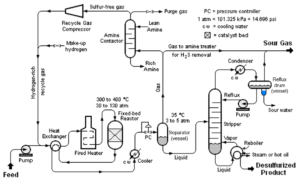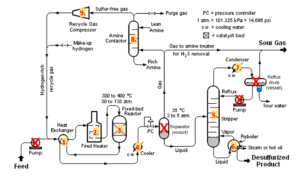# 48 Cost of Operating Labour

Learning Objectives

By the end of this section, you should be able to:

Understand the components of manufacturing costs

Estimate the cost of operating labour

## Manufacturing Costs

Typically divided into three main elements:

1. Direct manufacturing costs: Factors that vary directly with the rate of production
e.g. raw materials, plant operators
2. Fixed manufacturing costs: Factors not affected by the level of production, but related to manufacturing
e.g. taxes and insurance
3. General expenses: Management and administrative expenses not related to manufacturing

$\text{Cost of Manufacturing }(COM)=\text{direct cost} + \text{fixed cost} + \text{general expenses}$

## Useful Entities for Estimating Costs

• Fixed capital investment ($FCI$): We saw this previously and saw how to estimate this using the Lang factor and purchased equipment cost.
• Cost of raw materials ($CRM$) : We saw how to estimate these previously using chemical pricing data (recall estimating GEP).
• Cost of utilities ($C_{UT}$): Previously saw how to calculate our utility needs for a unit and assess costs associated with this (recall from the energy balance section).
• Cost of operating Labor ($C_{OL}$): Cost of operators – we will see how to estimate this.
• Cost of waste treatment ($C_{WT}$): Harder to estimate – there are ways to assess this, but it is beyond the scope of this class. For now, we will need to be given this for a process.

## Number of Operating Labour

$N_{OL}$ – Number of operators needed in the plant at a given time to ensure it runs smoothly.

The number of operators ($N_{OL}$) needed per shift can be estimated from a correlation by Alkhayat and Gerrard$^{}$ which is based on data from five major chemical companies.

$N_{OL}=(6.29+31.7P^2+0.23N_{np})^{0.5}$

where

$N_{OL}$ – number of operating labour workers at a given time

$P$ – process steps involving handling solids (transport, partical size control, particulate removal)

$N_{np}$ – number of process steps not invloving handling solids, including compression, heating, cooling, mixing, separation and reaction, but not including pumps and vessels

We will take the convention of rounding up to the nearest integer to ensure there is enough labour to operate the plant, although in practice there may be ways to have part-time staff etc.

## Cost of Operating Labour

$\text{# of operator}=N_{OL}×\frac{\text{# of total shifts in a year}}{\text{# of shifts per operator}}$

The number of operators is rounded up to the nearest integer when the calculation does not result in a whole number.

$\text{cost of operating labour}(C_{OL})=\text{# of operator}×\text{salary}$

The number of total shifts in a year and the number of shifts per operator can be calculated as follows:

On average an operator works five eight-hour shifts per week for 49 weeks per year (3 weeks off for sick leave and vacation deducted from 52 total weeks in a year).

$\text{# of shifts per operator} = 5 \frac{shifts}{week}×49\frac{weeks}{year}=245\text{ shifts}$

A plant generally requires operators 365 days a year including maintenance and shutdowns. One day is split into 3 eight-hour shifts.

$\text{# of total shifts in a year}= 365 days× 3 \frac{shifts}{day}=1095 \text{ operating shifts}$

The number of operators needed to fill a position that needs one operator at a given time is $\frac{1095}{245}≈4.5$.

To find the total cost of operating labour, we can use an average salary for a plant operator. The average salary for a plant operator can be found from an agency such as Statistics Canada.

Suppose each shift requires 4 operators on the site ($N_{OL}=4$), we can calculate the total cost of operating labour:

$\text{# of operator}=N_{OL}×\frac{\text{# of total shifts in a year}}{\text{# of shifts per operator}}=4×4.5=18$

The number should be rounded up if it does not result in a whole number.

Say an operator receives a salary of 70,000 dollars per year, the total cost of operating labour is $18×70,000=1,260,000$.

Exercise: Calculating the Cost of Operating Labour

A typical catalytic hydrodesulfurization process used in oil refineries to convert organic sulfur compounds in various process streams into hydrogen sulfide gas is shown below. All streams in the process only involve liquid and gaseous phases.Image obtained from mbeychok via Wikimedia commons / CC BY-SA 3.0

Given the information:

• On average, a worker in this plant works 5 shifts a week, 49 weeks a year
• The plant operates 365 days a year with 3 shifts per day
• The average salary for an operator in this plant is 80,000 dollars per year

Calculate the total cost of operating labour per year.

### Solution

Step 1: Calculate the number of operating labour ($N_{OL}$):

From the labels, the process does not involve handling solids($P=0$). And we can count that we have a total of 9 process steps that handle fluids without accounting for the number of pumps and vessels. This is shown in the diagram below:So $P=0\;;N_{np}=9$. Substituting this into the formula for the number of operators:

\begin{align*}
N_{OL}&=(6.29+31.7×(0)^2+0.23×9)^{0.5}\\
& = 2.89\\
& ≈ 3
\end{align*}

Therefore, each shift needs 3 operators.

Step 2: Calculate the number of operators needed to fill a position that needs one operator at a time:

$\text{# of shifts per operator per year} = 5 \frac{shifts}{week}×49\frac{weeks}{year}=245\frac{shifts}{year}$
$\text{# of total shifts in a year}= 365 days× 3 \frac{shifts}{day}=1095 \frac{shifts}{year}$
$\frac{\text{# of total shifts in a year}}{\text{# of shifts per operator per year}}=\frac{1095}{245}≈4.5$

Step 3: Calculate the total number of operators needs to be hired for the plant:

\begin{align*}
\text{# of operator} & =N_{OL}×\frac{\text{# of total shifts in a year}}{\text{# of shifts per operator}} \\
& =3×4.5\\
& = 13.5 \\
& ≈ 14
\end{align*}

Step 4: Calculate the total cost of operating labours:
\begin{align*}
C_{OL} & =\text{# of operators}×\text{salary} \\
& =14×$80,000\\ & =$1,120,000
\end{align*}

## References

 Alkhayat, W. A., and A. M. Gerrard, Estimating Manning Levels for Process Plants, AACE Transactions, I.2.1–I.2.4, 1984.